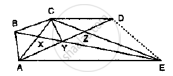# In Fig. Below, Cd || Ae and Cy || Ba. (I) Name a Triangle Equal in Area of δCbx (Ii) Prove that Ar (δ Zde) = Ar (δCza) (Iii) Prove that Ar (Bczy) = Ar (δ Edz) - Mathematics

In Fig. below, CD || AE and CY || BA.
(i) Name a triangle equal in area of ΔCBX
(ii) Prove that ar (Δ ZDE) = ar (ΔCZA)
(iii) Prove that ar (BCZY) = ar (Δ EDZ)#### Solution

Since, ΔBCA and  ΔBYAare on the same base BA and between same parallels BA and CY Then area (ΔBCA) = ar (BYA)

⇒ ar (Δ CBX) + ar (Δ BXA) = ar (Δ BXA) + ar (ΔAXY)

⇒ ar (Δ CBX)  = ar (Δ AXY)         ........ (1)

Since, ΔACE and ΔADE are on the same base AE and between same parallels CD and AE

⇒ (ΔCLA) + ar (ΔAZE) = ar (ΔAZE) + ar (ΔDZE)

⇒ ar (ΔCZA) = (ΔDZE)        ........ (2)

Since  ΔCBY and ΔCAY  are on the same base CY and between same parallels
BA and CY

Then ar  (ΔCBY)  ar (ΔCAY )
Adding ar (ΔCYG) on both sides, we get

⇒ ar (ΔCBX) + ar (ΔCYZ) = ar (ΔCAY) + ar (ΔCYZ)

⇒ ar (BCZX) = ar (Δ CZA)       ......... (3)

Compare equation (2) and (3)
ar (BCZY)  = ar (ΔDZE)

Concept: Corollary: A rectangle and a parallelogram on the same base and between the same parallels are equal in area.
Is there an error in this question or solution?

#### APPEARS IN

RD Sharma Mathematics for Class 9
Chapter 14 Areas of Parallelograms and Triangles
Exercise 14.3 | Q 21 | Page 47

Share